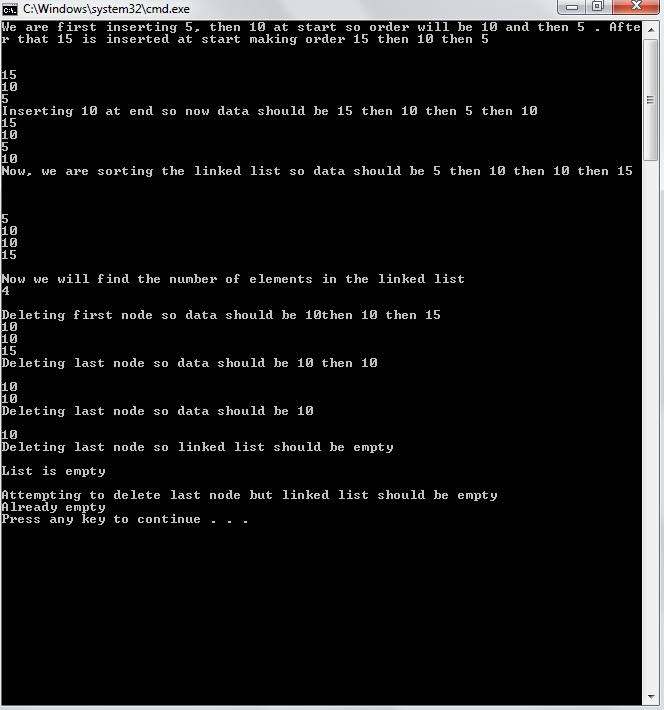# Linked List using C++ part4

`int count()	{		int count=0;		Node *cur;		cur=head;		while(cur!=NULL)		{			cur=cur->next;			count++;		}		return count;	}`
`void sort()	{		Node *cur= head;		int temp;		int counter=count();		for (int i=0; i<counter; i++)		{			cur = head;			while (cur->next!=NULL)			{				if (cur->data > cur->next->data)				{					temp=cur->data;					cur->data=cur->next->data;					cur->next->data=temp;					cur=cur->next;				}				else 					cur = cur->next;			}			}	}`
`~Linklist ()	{		while(!checkempty())		{			DeleteFirstNode();		}	}`
Here we delete every node one by one until the linked list is empty. Note: C++ codes along merged with the previous linked list codes are given along with these. In next tutorial , I will try to wind up basic functions of linked list like insertion in middle, deletion from middle and copy constructor. Output: